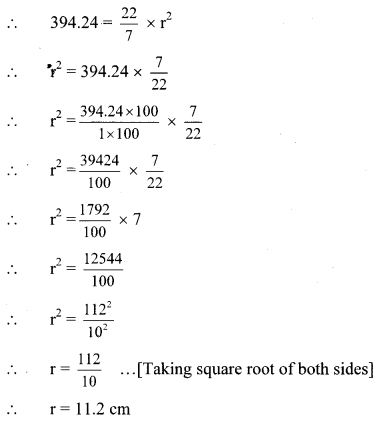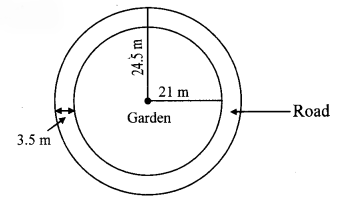# Maharashtra Board 8th Class Maths Practice Set 15.6 Solutions Chapter 15 Area

Balbharti Maharashtra State Board Class 8 Maths Solutions covers the Practice Set 15.6 8th Std Maths Answers Solutions Chapter 15 Area.

## Practice Set 15.6 8th Std Maths Answers Chapter 15 Area

Question 1.
Radii of the circles are given below, find their areas.
i. 28 cm
ii. 10.5 cm
iii. 17.5 cm
Solution:
i. Radius of the circle (r) = 28 cm … [Given]
Area of the circle = πr²
= $$\frac { 22 }{ 7 }$$ x (28)²
= $$\frac { 22 }{ 7 }$$ x 28 x 28
= 22 x 4 x 28
= 2464 sq. cm

ii. Radius of the circle (r) = 10.5 cm … [Given]
Area of the circle = πr²
= $$\frac { 22 }{ 7 }$$ x (10.5)²
= $$\frac { 22 }{ 7 }$$ x 10.5 x 10.5
= 22 x 1.5 x 10.5
= 346.5 sq. cm

iii. Radius of the circle (r) = 17.5 cm … [Given]
Area of the circle = πr²
= $$\frac { 22 }{ 7 }$$ x(17.5)²
= $$\frac { 22 }{ 7 }$$ x 17.5 x 17.5
= 22 x 2.5 x 17.5
= 962.5 sq. cm

Question 2.
Areas of some circles are given below, find their diameters.
i. 176 sq.cm
ii. 394.24 sq. cm
iii. 12474 sq. cm
Solution:
i. Area of the circle =176 sq. cm .. .[Given]
Area of the circle = πr²
∴ 176 = $$\frac { 22 }{ 7 }$$ x r²
∴ r² = 176 x $$\frac { 7 }{ 22 }$$
∴ r² = 56
∴ r = √56 … [Taking square root of both sides]
Diameter = 2r = 2√56 CM

ii. Area of the circle = 394.24 sq. cm … [Given]
Area of the circle = πr²∴ Diameter = 2r = 2 x 11.2 = 22.4 cm

iii. Area of the circle = 12474 sq. cm …[Given]
Area of the circle = πr²
∴ 12474 = $$\frac { 22 }{ 7 }$$ x r²
∴ r² = 12474 x $$\frac { 7 }{ 22 }$$
∴ r² = 567 x 7
∴ r² = 3969
∴ r = 63 …[Taking square root of both sides]
∴ Diameter = 2r = 2 x 63 = 126cm

Question 3.
Diameter of the circular garden is 42 m. There is a 3.5 m wide road around the garden. Find the area of the road.Solution:Diameter of the circular garden is 42 m. … [Given]
∴ Radius of the circular garden (r) = $$\frac { 42 }{ 2 }$$ = 21 m
Width of the road = 3.5 m …[Given]
Radius of the outer circle (R)
= 21 + 3.5
= 24.5 m
Area of the road = area of outer circle – area of circular garden
= πR² – πr²
= π (R² – r²)
= $$\frac { 22 }{ 7 }$$ [(24.5)² – (21)²]
= $$\frac { 22 }{ 7 }$$ (24.5 + 21) (24.5 – 21)
…..[∵ a²-b² = (a+b)(a-b)]
= $$\frac { 22 }{ 7 }$$ x 45.5 x 3.5
= 22 x 45.5 x 0.5
= 500.50 sq. m
∴ The area of the road is 500.50 sq. m.

Question 4.
Find the area of the circle if its circumference is 88 cm.
Solution:
Circumference of the circle = 88 cm …[Given]
Circumference of the circle = 2πr
∴ 88 = 2 x $$\frac { 22 }{ 7 }$$ x r
∴ $$r=\frac{88 \times 7}{2 \times 22}$$ ∴ r = 14cm
Area of the circle = πr² = $$\frac { 22 }{ 7 }$$ x (14)²
= $$\frac { 22 }{ 7 }$$ x 14 x 14 = 22 x 2 x 14 = 616 sq. cm
∴ The area of circle is 616 Sq cm

Maharashtra Board Class 8 Maths Chapter 15 Area Practice Set 15.6 Intext Questions and Activities

Question 1.
Draw a circle of radius 28mm. Draw any one triangle and draw a trapezium on the graph paper. Find the area of these figures by counting the number of small squares on the graph paper. Verify your answers using formula for area of these figures.
Observe that smaller the squares of graph paper, better is the approximation of area. (Textbook pg. no. 105)
Solution:
(Students should do this activity on their own.)